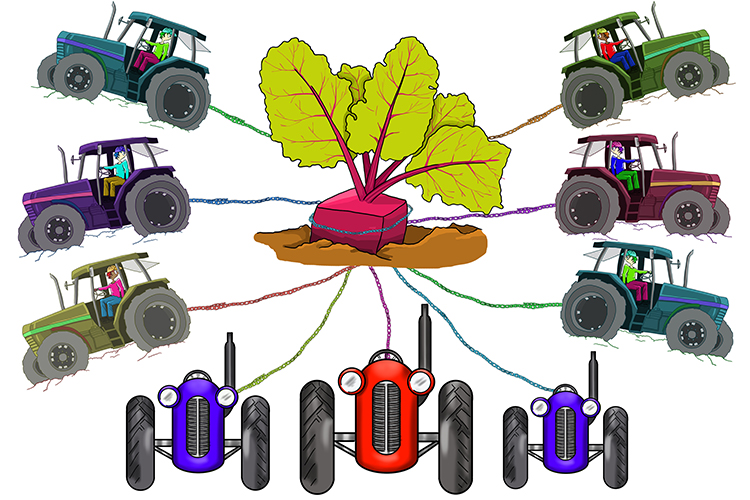# How to remember

Think of sqrt9“That’s absurd!” (surd) Surely you can remove a square beetroot.” But they couldn’t remove it (cant remove). “We need more lines, perhaps nine.”

## Surd examples

sqrt7+4\sqrt7

This is really asking us to add 1sqrt7+4sqrt7

Try something we know in sqrt9

sqrt9+4sqrt9=3+4times3=15

Is that the same as

5sqrt9=15

Yes it is

Therefore  1sqrt7+4sqrt7

=5sqrt7

2.  Subtract these surds

5sqrt2-3sqrt2

Try something we know in sqrt9

5sqrt9 -3sqrt9

=5times3-3times3=15-9=6

Is that the same as

(5-3)sqrt9=6

Yes it is

Therefore  5sqrt2-3sqrt2

= (5-3)sqrt2

= 2sqrt2

4 sqrt2+3sqrt3

These cannot be added as these surds are not the same.

It’s not in our rules.

4.  Work out

2sqrt2+3sqrt2-sqrt2

Try something we know in sqrt9

2sqrt9+3sqrt9-1sqrt9

=2times3+3times3-3

=6+9-3=12

Is that the same as

(2+3-1)sqrt9=12

Yes it is

Therefore  2sqrt2+3sqrt2-1sqrt2

=(2+3-1)sqrt2

=4sqrt2

5.  Simplify

sqrt8/sqrt2

sqrt9/sqrt9=3/3=1=sqrt(9/9)

Therefore  sqrt8/sqrt2=sqrt(8/2)=sqrt4=2

Answer:  sqrt8/sqrt2=2

6.  Simplify

sqrt2timessqrt3

Try something we know in sqrt9

sqrt4timessqrt9=2times3=6

Is this the same as

sqrt{4times9}= sqrt36=6

Yes it is

Thereforesqrt2timessqrt3=sqrt{2times3}=sqrt6

Answer:  sqrt2timessqrt3=sqrt6

7.  Simplify

sqrt12

sqrt9timessqrt9=3times3=9

=sqrt81=sqrt{9times9}

With this example we can see

sqrt12=sqrt{3times4}=sqrt3 timessqrt4

sqrt3timessqrt4=sqrt3times2=2sqrt{3

Answer:  sqrt12=2sqrt3

8.  Simplify

sqrt15/sqrt5

Write down what we know

sqrt9/sqrt9=3/3=1=sqrt(9/9)

Therefore  sqrt9/sqrt9=sqrt(9/9)

Therfore sqrt15/sqrt5=sqrt(15/5)=sqrt3

Answer:  sqrt15/sqrt5=sqrt3

9.  Simplify

2sqrt3+sqrt2-4sqrt3

Rearrange

2sqrt3-4sqrt3+sqrt2

Try something we know

2timessqrt9-4sqrt9

=2times3-4times3=6-12=-6

Is that the same as

(2-4)sqrt9=-2times3=-6

Yes it is

Therefore  2sqrt3-4sqrt3+sqrt2

=(2-4)sqrt3+sqrt2

=-2sqrt3+sqrt2

Answer:  2sqrt3+sqrt2-4sqrt3=-2sqrt3+sqrt2

10.  Simplify

5sqrt2times2sqrt3

Try something we know in sqrt9

5sqrt9times2sqrt9=5times3times2times3

=15times6=90

Is this the same as

5times2sqrt(9times9)=10timessqrt81

=10times9=90

Yes it is

Therefore  5timessqrt2times2timessqrt3

= 5times2timessqrt2timessqrt3

= 10timessqrt2timessqrt3

= 10timessqrt(2times3)

= 10timessqrt6

Answer:  5sqrt2times2sqrt3=10sqrt6